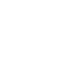304 North Cardinal St.
Dorchester Center, MA 02124

# 使用 GSAP 的位置參數 (position parameter) 建立更有彈性的時間軸

## GSAP 3 的基本時間軸語法

```// 實體化時間軸
const tl = gsap.timeline();

// 基礎語法

// 在 to 方法中加入 position 參數

See the Pen GSAP Position Parameter Test by Eric Chuang (@eric-chuang) on CodePen.

### “+=秒數” “-=秒數”

See the Pen GSAP Position Parameter += -= by Eric Chuang (@eric-chuang) on CodePen.

### 透過 “<” 貼齊前一個時間軸的起始時間

```/** 情況一: Circle 與 Triangle 會同時 */
tl.to( square, {duration: 1, x: 400} )
.to( circle, {duration: 1, x: 400}, "+=1" )
.to( triangle, {duration: 1, x: 400}, "-=1" );

/** 情況二: Square, Circle 與 Triangle 會同時 */
tl.to( square, {duration: 1, x: 400} )
.to( circle, {duration: 1, x: 400}, "-=1" )
.to( triangle, {duration: 1, x: 400}, "-=1" );```

```/** 情況一: Circle 與 Triangle 會同時 */
tl.to( square, {duration: 1, x: 400} )
.to( circle, {duration: 1, x: 400}, "+=1" )
.to( triangle, {duration: 1, x: 400}, "<" );

/** 情況二: Square, Circle 與 Triangle 會同時 */
tl.to( square, {duration: 1, x: 400} )
.to( circle, {duration: 1, x: 400}, "<" )
.to( triangle, {duration: 1, x: 400}, "<" );

/** 情況三: Square 與 Circle 同時，而 Triangle 會過一秒後執行 */
tl.to( square, {duration: 1, x: 400} )
.to( circle, {duration: 1, x: 400}, "<" )
.to( triangle, {duration: 1, x: 400}, "<+1" );```

### 透過數字，指定動畫發生的絕對位置

```/** 指定絕對位置 */
tl.to( square, {duration: 1, x: 400} )
.to( circle, {duration: 1, x: 400}, 3 )
.to( triangle, {duration: 1, x: 400}, 5 );

/** 相當於以下的相對位置 */
tl.to( square, {duration: 1, x: 400} )
.to( circle, {duration: 1, x: 400}, "<+1" )
.to( triangle, {duration: 1, x: 400}, "<+1" );```

### 透過標籤製作關鍵影格

```時間軸.to( 選擇器, {屬性值} )
.to( 選擇器2, {屬性值}, "關鍵影格名稱" )
.to( 選擇器3, {屬性值}, "關鍵影格名稱+=/-=相對時間" );```

```tl.to( square, {duration: 1, x: 400} )
.to( circle, {duration: 1, x: 400}, "group1" )
.to( triangle, {duration: 1, x: 400}, "group1" );```

See the Pen GSAP Position Parameter Tag by Eric Chuang (@eric-chuang) on CodePen.

## 參考資料